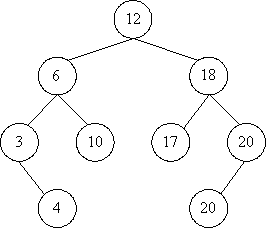Online JudgeProblem SetAuthorsOnline ContestsUser
Web Board
F.A.Qs
Statistical Charts
Problems
Submit Problem
Online Status
Prob.ID:
Register
Authors ranklist
Current Contest
Past Contests
Scheduled Contests
Award Contest
Register
Language:
The Number of the Same BST
 Time Limit: 1000MS Memory Limit: 65536K Total Submissions: 2854 Accepted: 846

Description

Many people knows binary search tree. The keys in a binary search tree are always stored in such a way as to satisfy the BST property:

Let x be a node in a binary search tree. If y is a node in the left subtree of x, then key[y] <= key[x]. If y is a node in the right subtree of x, then key[y] > key[x].

For example,It is a binary search tree. And it can be built by inserting the elements of vector A <12, 6, 3, 18, 20, 10, 4, 17, 20> sequentially. But it can also be built by the vector B <12, 18, 17, 6, 20, 3, 10, 4, 20>.

Now given a vector X, then you may get a binary search tree from X. Your job is to calculate how many different vectors can build the same binary search tree. To make it easy, you should just output the number of different vectors mod 9901.

Input

Input consists of several cases. Each case starts with a line containing one positive integer n, which is the length of test vector. The integer n is less than 100. Following this there will be n positive integers, which are less then 10000, on the next line. The input will end with a case starting with n = 0. This case should not be processed.

Output

For each test case, print a line with a single integer, which is the number of different vectors mod 9901.

Sample Input

```3
2 1 3
9
5 6 3 18 20 10 4 17 20
0
```

Sample Output

```2
168
```

Source

[Submit]   [Go Back]   [Status]   [Discuss]Home PageGo BackTo top